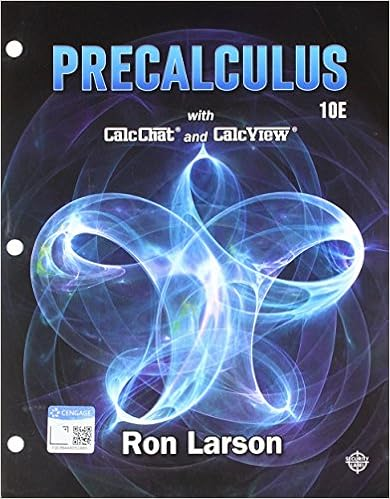# Edmundscom would like to test the hypothesis that the

• 56
• 93% (30) 28 out of 30 people found this document helpful

This preview shows page 30 - 32 out of 56 pages.

##### We have textbook solutions for you!
The document you are viewing contains questions related to this textbook.The document you are viewing contains questions related to this textbook.
Chapter 3 / Exercise 80
Precalculus
Hostetler/LarsonExpert Verified
80)Edmunds.com would like to test the hypothesis that the standard deviation for the age of animported car on the road is greater than the standard deviation for the age of a domestic car. Thefollowing data shows the ages of a random sample of import and domestic cars.Import13454510Domestic4546546The standard deviation for the age of domestic cars would be ________.80)A)2.15B)0.90C)5.03D)3.78Answer:B
Explanation:A)B)C)D)81)The Canadian government would like to test the hypothesis that the standard deviation for thehourly wage for men is equal to the standard deviation for the hourly wage for women. Thefollowing data summarizes the sample statistics for hourly wages for men and women. MenWomenSample standard deviation\$6.10\$6.80Sample size2125Using ΅=0.05, the critical value for this hypothesis test would be ________.81)
Explanation:A)B)C)D)30
##### We have textbook solutions for you!
The document you are viewing contains questions related to this textbook.The document you are viewing contains questions related to this textbook.
Chapter 3 / Exercise 80
Precalculus
Hostetler/LarsonExpert Verified
82)A retail chain would like to test if a difference exists between the variability of customer satisfactionscores from two locations. The following data represents satisfaction scores from a random sampleof customers from these locations on a scale of 1-20.Location 112142018151716Location 2181917181620The variance for the satisfaction scores at Location 2 would be ________.82)
Explanation:A)B)C)D)83)A professor would like to test the hypothesis that the average number of minutes that a studentneeds to complete a statistics exam has a standard deviation that is less than 5.0 minutes. A randomsample of 15 students was selected and the sample standard deviation for the time needed tocomplete the exam was found to be 4.0 minutes. The degrees of freedom for this hypothesis testwould be ________.83)
Explanation:A)B)C)D)84)A golfer would like to test the hypothesis that the variance of his golf score equals 10.0. A randomsample of 28 rounds of golf had a sample standard deviation of 3.5. Using ΅=0.10, the conclusionfor this hypothesis test would be that because the test statistic is ________________.84)A)more than the upper critical value, we can reject the null hypothesis and conclude that thevariance for this golfer's scores is not equal to 10.0B)less than the lower critical value, we fail to reject the null hypothesis and conclude that thevariance for this golfer's scores is not equal to 10.0C)between the lower and upper critical values, we reject the null hypothesis and cannotconclude that the variance for this golfer's scores is not equal to 10.0D)between the lower and upper critical values, we fail to reject the null hypothesis and cannotconclude that the variance for this golfer's scores is not equal to 10.0Answer:A
Explanation:A)B)C)D)31
•••